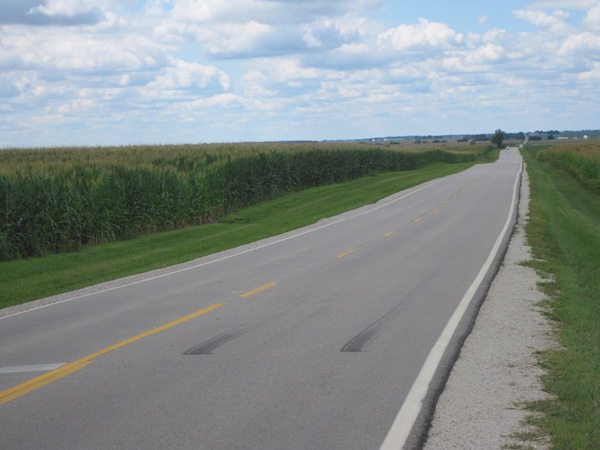## DistanceBasics
The formula provided for distance by the Mathematics Formula Sheet is straightforward, but it can be rearranged to ask questions about rate and time, too.

A question involving distance is often a plug-in (where you end up plugging in numbers for letters).

The word per is a hint that a distance-related question could be coming.

distance = rate × time
d = rt
d = distance
r = rate
t = time

Formulas (provided by Mathematics Formula Sheet)

d = rt

Formulas (NOT provided by Mathematics Formula Sheet)

r = d ÷ t

t = d ÷ r

Question

A car traveled at a speed of 55 miles per hour for 9 hours.
What distance did it travel?

495 miles

r = 55 miles per hour
t = 9 hours
d = rt
d = 55 × 9 = 495

Calculator Click What You See Commentblinker clears screen
55 × 9 55*9 d = rt495 Answer
###### Distance

Question
A car traveled 715 miles in 11 hours.
What was the car’s speed in miles per hour?

65 miles per hour

d = 715 miles
t = 11 hours
r = d ÷ t
r = 715 ÷ 11 = 65

Calculator Click What You See Commentblinker clears screen
715 ÷ 11 715÷11 r = d ÷ t65 Answer
###### Distance: Rate

Question
A car traveled 980 miles at a speed of 70 miles per hour.
How much time elapsed?

14 hours

d = 980 miles
r = 70 miles per hour
t = d ÷ r
t = 980 ÷ 70 = 14

Calculator Click What You See Commentblinker clears screen
980 ÷ 70 980÷70 t = d ÷ r14 Answer
###### Distance: Time

Practice – Questions

1.  A car traveled at a speed of 75 kilometers per hour for 11 hours.
What distance did it travel?

2.  A rocket traveled at a speed of 17555 miles per hour for 24 hours.
What distance did it travel?

3.  A car traveled 1120 kilometers in 14 hours.
What was the car’s speed in kilometers per hour?

4.  A rocket traveled 669600 miles in 36 hours.
What was the rocket’s speed in miles per hour?

5.  A car traveled 650 miles at a speed of 50 miles per hour.  How much time elapsed?